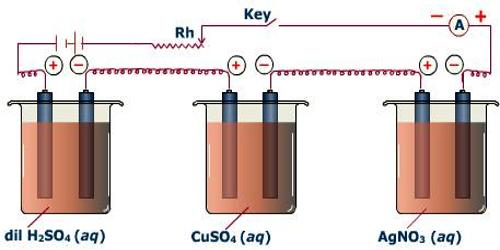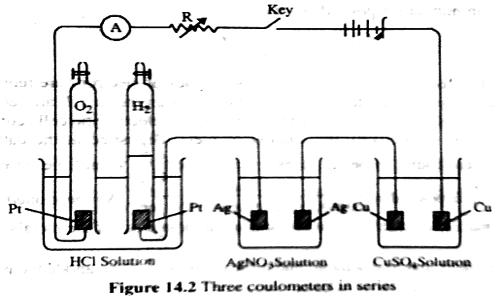Chemistry

# Proof of the Second Law of FaradayProof of the Second Law of Faraday

Second Law: The mass of a substance liberated at an electrode by a given amount of charge is proportional to the *chemical equivalent of the substance. That means, when the same quantity of electricity is passed through several electrolytes, the mass of the substances deposited are proportional to their respective chemical equivalent or equivalent weight.

The second law can be verified by using solutions of HCl, AgNO3 and CuSO4 in an apparatus as shown in Figure which consists of three coulometers arranged in series. In all cases the cathode used is of platinum (an inert metal). The same amount of current is passed for a definite time, and the amount of positive ions liberated at the cathode in each coulometer is determined. It is found that the amount discharged is in the proportion of half mole of hydrogen: one mole of silver; half mole of copper, i.e., to 1 : 107.88 : 31.78 (which were regarded as their respective equivalent masses). This verifies the second law. The discharge reactions are

H+ + e= ½ H2; Ag+ + e = Ag; ½ Cu2+ + e = ½ Cu